# thesIt

##Arif

• #### 12:41:37 pm on August 22, 2010 | # | 0 Tags: cross-validation, error, estimation, k-fold, Michaelsen 1987, reference, Stone 1974

Stone 1974 is referenced in:
Michaelsen J. 1987. Cross-validation in statistical climate forecast models. J Climate Applied Meteorology, 26:1589-1600

1520-0450(1987)026-1589-cviscf-2.0.co;2.pdf

Set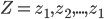$Z = z_{1}, z_{2},... , z_{1}$ consists of predictions and targets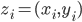$z_{i}=(x_{i}, y_{i})$

A set of prediction rule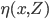$\eta (x,Z)$ will be used to predict y0 from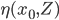$\eta (x_{0},Z)$

Let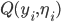$Q(y_{i},\eta_{i})$ be the accuracy.
by least squares this will usually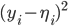$(y_{i}-\eta_{i})^{2}$
in other words expected Err is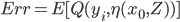$Err= E[Q(y_{i},\eta(x_{0},Z))]$

## MSE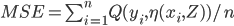$MSE= \sum_{i=1}^{n}Q(y_{i},\eta(x_{i},Z))/n$

In cross validation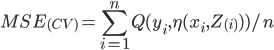$MSE_{(CV)}= \sum_{i=1}^{n}Q(y_{i},\eta(x_{i},Z_{(i)}))/n$